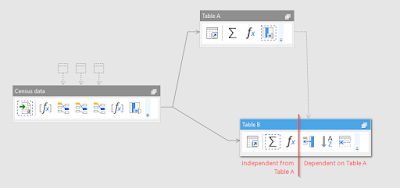## Nov 30, 2016

### Using Synchronize transformation

In order to speed up calculations EasyMorph automatically attempts to execute transformations in independent tables in parallel. Here independent means that transformations in one table don't depend on transformations in another tables. In the example below Table A is completely independent from Table B. Although, in Table B only the first three transformations are independent from Table A, because the 4th transformation (Peek) has dependency of Table A which makes all consequent transformations also dependent.click to zoom

After table 'Census data' is calculated, EasyMorph will start calculating transformations in Table A and Table B in parallel. However, since the Peek transformation in Table B depends on Table A (notice the dotted line from Table A to Peek), it will calculate the first 3 transformations in Table B simultaneously with Table A, then reach Peek and wait until calculation of Table A is finished. After that it will calculate Peek and all the remaining transformations in Table B.

While automatic parallelization is good for performance it may lead to conflicts in cases when transformations in independent tables attempt to modify the same external file at the same time. Most typically this happens when a project exports data into different sheets of the same spreadsheet. In order to synchronize export transformations (or other side-effect operations) in such cases the Synchronize transformation should be used. This transformation instructs EasyMorph to wait until another table is calculated. In another words, it inserts a dependency on another table.

Below is an example where three 1-column tables are exported into three sheets of the same spreadsheet. Notice Synchronize transformations inserted before export transformations in tables "Export to Sheet2" and "Export to Sheet3". They ensure that all export transformations are executed sequentially, even if they are in different tables.click to zoom